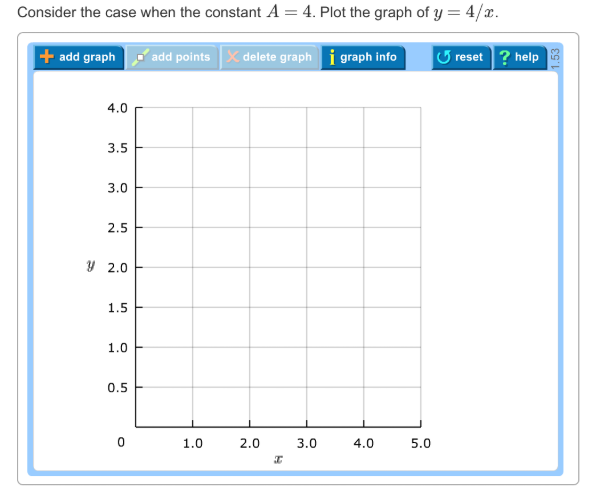# Problem: Consider the case when the constant A = 4. Plot the graph of y = 4/x.

###### FREE Expert Solution

At x = 1, y = 4/1 = 4

At x = 2, y = 4/2 = 2

At x = 3, y = 4/3 = 1.33

98% (310 ratings)###### Problem Details

Consider the case when the constant A = 4. Plot the graph of y = 4/x.